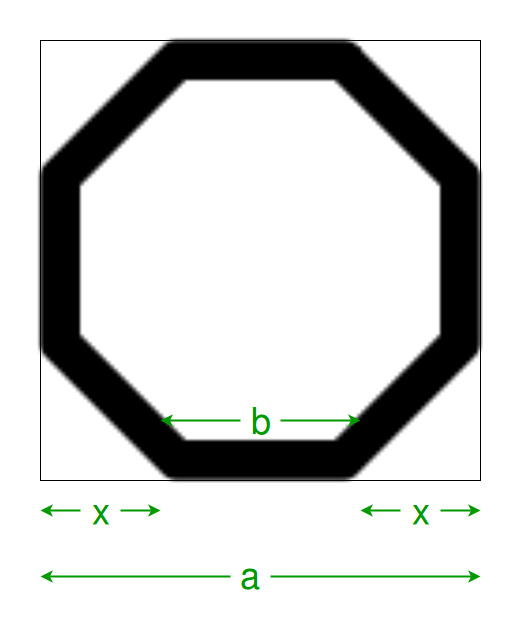# Program to find the side of the Octagon inscribed within the square

Given a square of side length ‘a’, the task is to find the side length of the biggest octagon that can be inscribed within it.

Examples:

```Input: a = 4
Output: 1.65685

Input: a = 5
Output: 2.07107
```

## Recommended: Please try your approach on {IDE} first, before moving on to the solution.Approach:

=> From the figure, it can be seen that, side length of the Octagon = b
=> Also since the polygons are regular, therefore 2*x + b = a
=> From the right angled triangle, x^2 + x^2 = b^2

=> Hence, x = b/√2,
=> So, √2b + b = a

=> Therefore, b = a/(√2 +1)

Below is the implementation of the above approach:

## C++

 `// C++ Program to find the side of the octagon ` `// which can be inscribed within the square ` ` `  `#include ` `using` `namespace` `std; ` ` `  `// Function to find the side ` `// of the octagon ` `float` `octaside(``float` `a) ` `{ ` ` `  `    ``// side cannot be negative ` `    ``if` `(a < 0) ` `        ``return` `-1; ` ` `  `    ``// side of the octagon ` `    ``float` `s = a / (``sqrt``(2) + 1); ` `    ``return` `s; ` `} ` ` `  `// Driver code ` `int` `main() ` `{ ` ` `  `    ``// Get he square side ` `    ``float` `a = 4; ` ` `  `    ``// Find the side length of the square ` `    ``cout << octaside(a) << endl; ` ` `  `    ``return` `0; ` `} `

## Java

 `// Java Program to find the side of the octagon  ` `// which can be inscribed within the square  ` ` `  `import` `java.io.*; ` ` `  `class` `GFG { ` `     `  `// Function to find the side  ` `// of the octagon  ` `static` `double` `octaside(``double` `a)  ` `{  ` ` `  `    ``// side cannot be negative  ` `    ``if` `(a < ``0``)  ` `        ``return` `-``1``;  ` ` `  `    ``// side of the octagon  ` `    ``double` `s = a / (Math.sqrt(``2``) + ``1``);  ` `    ``return` `s;  ` `}  ` ` `  `// Driver code  ` `     `  `    ``public` `static` `void` `main (String[] args) { ` `         `  `    ``// Get he square side  ` `    ``double` `a = ``4``;  ` ` `  `    ``// Find the side length of the square  ` `    ``System.out.println( octaside(a));  ` ` `  `         `  `         `  `    ``} ` `} ` `//This Code  is contributed by ajit `

## Python3

 `# Python 3 Program to find the side  ` `# of the octagon which can be  ` `# inscribed within the square ` `from` `math ``import` `sqrt ` ` `  `# Function to find the side ` `# of the octagon ` `def` `octaside(a): ` `     `  `    ``# side cannot be negative ` `    ``if` `a < ``0``: ` `        ``return` `-``1` ` `  `    ``# side of the octagon ` `    ``s ``=` `a ``/` `(sqrt(``2``) ``+` `1``) ` `    ``return` `s ` ` `  `# Driver code ` `if` `__name__ ``=``=` `'__main__'``: ` `     `  `    ``# Get he square side ` `    ``a ``=` `4` ` `  `    ``# Find the side length of the square ` `    ``print``(``"{0:.6}"``.``format``(octaside(a))) ` `     `  `# This code is contributed ` `# by Surendra_Gangwar `

## C#

 `// C# Program to find the side  ` `// of the octagon which can be  ` `// inscribed within the square  ` `using` `System; ` ` `  `class` `GFG ` `{ ` `     `  `// Function to find the side  ` `// of the octagon  ` `static` `double` `octaside(``double` `a)  ` `{  ` ` `  `    ``// side cannot be negative  ` `    ``if` `(a < 0)  ` `        ``return` `-1;  ` ` `  `    ``// side of the octagon  ` `    ``double` `s = a / (Math.Sqrt(2) + 1);  ` `    ``return` `s;  ` `}  ` ` `  `// Driver code  ` `static` `public` `void` `Main () ` `{ ` `    ``// Get he square side  ` `    ``double` `a = 4;  ` `     `  `    ``// Find the side length  ` `    ``// of the square  ` `    ``Console.WriteLine( octaside(a));  ` `}  ` `}  ` ` `  `// This code is contributed  ` `// by akt_mit `

## PHP

 ` `

Output:

```1.65685
```

Attention reader! Don’t stop learning now. Get hold of all the important DSA concepts with the DSA Self Paced Course at a student-friendly price and become industry ready.

My Personal Notes arrow_drop_upBudding Web DeveloperKeen learnerAverage CoderDancer&Social Activist

If you like GeeksforGeeks and would like to contribute, you can also write an article using contribute.geeksforgeeks.org or mail your article to contribute@geeksforgeeks.org. See your article appearing on the GeeksforGeeks main page and help other Geeks.

Please Improve this article if you find anything incorrect by clicking on the "Improve Article" button below.

Improved By : jit_t, SURENDRA_GANGWAR

Article Tags :
Practice Tags :

Be the First to upvote.

Please write to us at contribute@geeksforgeeks.org to report any issue with the above content.